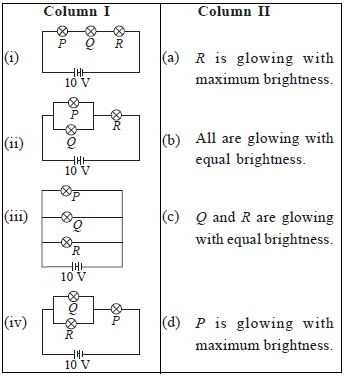# User Forum

Subject :NSO    Class : Class 7

In column I, three identical lamps P, Q and R are arranged in different configurations, while in column II the information about brightness of lamps are mentioned. Match column I with column II and select the correct option from the given codes. (Neglect resistance of connecting wires)A (i) → (A, C), (ii) → (A), (iii) → (B, C), (iv) → (C, D)
B (i) → (C, D), (ii) → (B), (iii) → (B, D), (iv) → (A, B)
C (i) → (A), (ii) → (C, D), (iii) → (B, C), (iv) → (B)
D (i) → (B, D), (ii) → (C), (iii) → (B, C), (iv) → (A)